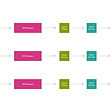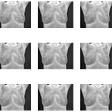# Solving Sudoku Programmatically

Terminology:

• Boxes are individual squares
• Units are complete rows, columns, and 3x3 squares
• Peers are boxes that belong to the same unit
`rows = 'ABCDEFGHI'cols = '123456789'def cross(a, b):    # output = []    #     for s in a:    #         for t in b:    #             output.append(s+t)    # return output    return [s+t for s in a for t in b]boxes = cross(rows, cols)row_units = [cross(r, cols) for r in rows]column_units = [cross(rows, c) for c in cols]square_units = [cross(rs, cs) for rs in ('ABC','DEF','GHI') for cs in ('123','456','789')]unitlist = row_units + column_units + square_unitsunits = dict((s, [u for u in unitlist if s in u]) for s in boxes)peers = dict((s, set(sum(units[s],[]))-set([s])) for s in boxes)`

For unsolved boxes, eliminate solved peer box values from possible values:

`def eliminate(values):    for box, val in values.items():        if len(val) == 1:            for peer in peers[box]:                values[peer] = values[peer].replace(val, '')`

For unsolved boxes in a unit, if a possible digit appears once in a box, then that box is the only choice for that digit:

`def only_choice(values):    for unit in unitlist:        for digit in "123456789":            boxes = [box for box in unit if digit in values[box]]            if len(boxes) == 1:                values[boxes] = digit`

Repeatedly reduce possible solutions using these two methods, eliminate and only choice, until no progress is made:

`def reduce_puzzle(values):    progress = True    while progress:        # Check how many boxes have a determined value               solved_values_before = len([box for box in values.keys() if len(values[box]) == 1])        eliminate(values)        only_choice(values)        # Check how many boxes have a determined value, to compare        solved_values_after = len([box for box in values.keys() if len(values[box]) == 1])        # If no new values were added, stop the loop.        progress = solved_values_before != solved_values_after`

Once board is reduced as much as possible, get unsolved box with the least number of possible digits and try each digit until board is solved:

`def invalid(values):    return len([box for box in values.keys() if len(values[box]) == 0]) != 0def solved(values):    return len([box for box in values.keys() if len(values[box]) != 1]) == 0def search(values):    reduce_puzzle(values)    if invalid(values):        return None    if solved(values):        return values    n,s = min((len(values[s]), s) for s in boxes if len(values[s]) > 1)    for value in values[s]:        tmp = values.copy()        tmp[s] = value        tmp = search(tmp)        if tmp:            return tmp`

In Sudoku, by eliminating the possibilities in a given box we can eventually narrow down the board to the solution one by one. When solving Sudoku, mentally if there is a digit within a “peer” (horizontal row, vertical row, or 3x3 grid) we know that that digit cannot be repeated again. We also know that if amongst all the open possibilities in a peer, there is a unique digit, that digit must be the solution to that box (Example: If we have four boxes open and the possibilities for those boxes are 123, 234, 234, 24, then we know that from the first box’s possibilities of 123, that 1 is unique, so that can be the only possible solution to that box). By using these two techniques we can reduce the possibilities and partially solve the board.

After reducing the board down using those two techniques, we can further solve the board by trying a possibility for a box and seeing if that leads us down to a solution; if not, we must try the next possibility and so on.

The two parts to solving Sudoku can be referred to as constraint propagation and search.

--

--

--

## More from Paul Yeo

disscepolo della sperientia

Love podcasts or audiobooks? Learn on the go with our new app.

## Building a distributed MIDI Controller with Android Things and Nearby API## FAQ | Simon Says Extension for Final Cut Pro X## 60 Practices For Quality Engineering : Skills (Part 5)## Garbage Collection — Java How it works ?## Complete Guide to Whitelist Lottery & SolRazr IDO## Flipside’s Chainwalkers and The Redshift COPY Command## Paul Yeo

disscepolo della sperientia

## Breast Cancer Detection## Rectified Linear Activation Unit (ReLU)## Hard Drive Failure Detection using S.M.A.R.T attributes on Backblaze dataset.## How To Teach Machines To Learn Like Humans | Dataloop Blog